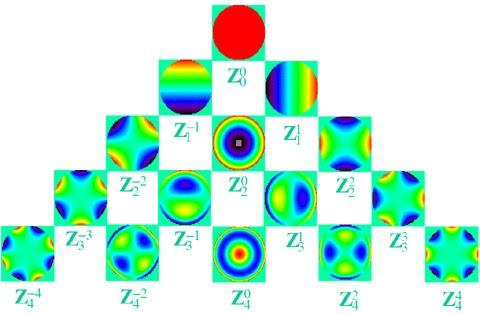# Problem 1000. Image Processing 004: Zernike Coefficients

Fit Zernike Terms to a wavefront.
The class notes of Schwiegerling UofA on ANSI Standard Zernike terms is the basis for this challenge. Pages 13 and 14 give the term definitions implemented in the zern_data_v01.m routine provided in the function template.ANSI Standard Zernike Chart:
Algorithm Description:
M=surface array to be fit by Zernike terms;
-valid data is circle of normalized radius<=1
Use the R and T arrays to create the A array
R=radial normalized distance from center of array
T=angular radian value from center of array
A=fitting array [valid_data_pts,terms]
Create A * z_fit = M(valid_data_pts)
[valid_pts,terms] * [terms,1] = [valid_pts,1]
Loop term=0:terms-1
- Create the 2-D array of z-terms uisng z=zern_data_v01(term,R,T)
- A(:,term+1) = z(valid_data_pts)
End Loop
Solve for z_fit: pinv(A)*M(valid_pts) or A\M(valid_pts)
Inputs: [M N R T] where N is number of Zernike terms for fit
Outputs: [v] Zernike fit vector of length N
Creation of the M surface and expected v fit vector can be seen in the Test Suite.

### Solution Stats

25.0% Correct | 75.0% Incorrect
Last Solution submitted on Oct 01, 2020

### Community Treasure Hunt

Find the treasures in MATLAB Central and discover how the community can help you!

Start Hunting!